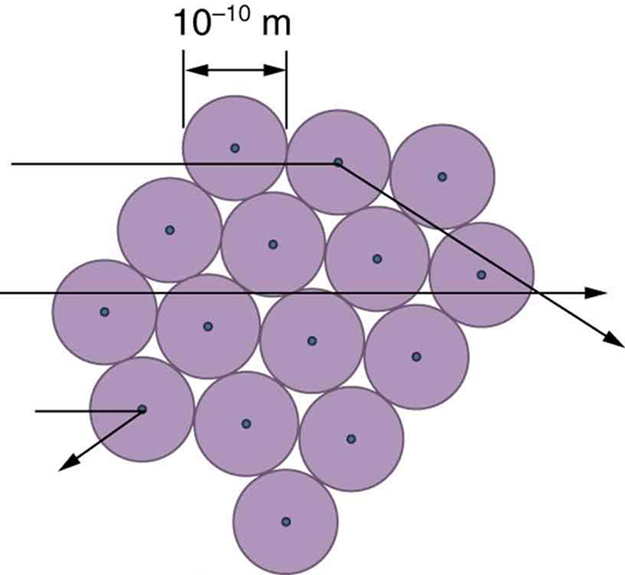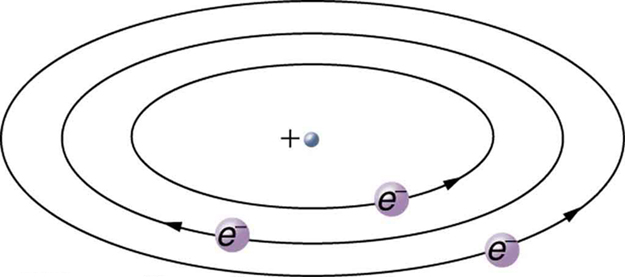# 30.2 Discovery of the parts of the atom: electrons and nuclei  (Page 5/7)

 Page 5 / 7

Although the results of the experiment were published by his colleagues in 1909, it took Rutherford two years to convince himself of their meaning. Like Thomson before him, Rutherford was reluctant to accept such radical results. Nature on a small scale is so unlike our classical world that even those at the forefront of discovery are sometimes surprised. Rutherford later wrote: “It was almost as incredible as if you fired a 15-inch shell at a piece of tissue paper and it came back and hit you. On consideration, I realized that this scattering backwards ... [meant] ... the greatest part of the mass of the atom was concentrated in a tiny nucleus.” In 1911, Rutherford published his analysis together with a proposed model of the atom. The size of the nucleus was determined to be about ${\text{10}}^{-\text{15}}\phantom{\rule{0.25em}{0ex}}\text{m}$ , or 100,000 times smaller than the atom. This implies a huge density, on the order of ${\text{10}}^{\text{15}}\phantom{\rule{0.25em}{0ex}}{\text{g/cm}}^{3}$ , vastly unlike any macroscopic matter. Also implied is the existence of previously unknown nuclear forces to counteract the huge repulsive Coulomb forces among the positive charges in the nucleus. Huge forces would also be consistent with the large energies emitted in nuclear radiation.

The small size of the nucleus also implies that the atom is mostly empty inside. In fact, in Rutherford’s experiment, most alphas went straight through the gold foil with very little scattering, since electrons have such small masses and since the atom was mostly empty with nothing for the alpha to hit. There were already hints of this at the time Rutherford performed his experiments, since energetic electrons had been observed to penetrate thin foils more easily than expected. [link] shows a schematic of the atoms in a thin foil with circles representing the size of the atoms (about ${\text{10}}^{-\text{10}}\phantom{\rule{0.25em}{0ex}}\text{m}$ ) and dots representing the nuclei. (The dots are not to scale—if they were, you would need a microscope to see them.) Most alpha particles miss the small nuclei and are only slightly scattered by electrons. Occasionally, (about once in 8000 times in Rutherford’s experiment), an alpha hits a nucleus head-on and is scattered straight backward.An expanded view of the atoms in the gold foil in Rutherford’s experiment. Circles represent the atoms (about 10 − 10 m size 12{"10" rSup { size 8{ - "10"} } " m"} {} in diameter), while the dots represent the nuclei (about 10 − 15 m size 12{"10" rSup { size 8{ - "15"} } " m"} {} in diameter). To be visible, the dots are much larger than scale. Most alpha particles crash through but are relatively unaffected because of their high energy and the electron’s small mass. Some, however, head straight toward a nucleus and are scattered straight back. A detailed analysis gives the size and mass of the nucleus.

Based on the size and mass of the nucleus revealed by his experiment, as well as the mass of electrons, Rutherford proposed the planetary model of the atom    . The planetary model of the atom pictures low-mass electrons orbiting a large-mass nucleus. The sizes of the electron orbits are large compared with the size of the nucleus, with mostly vacuum inside the atom. This picture is analogous to how low-mass planets in our solar system orbit the large-mass Sun at distances large compared with the size of the sun. In the atom, the attractive Coulomb force is analogous to gravitation in the planetary system. (See [link] .) Note that a model or mental picture is needed to explain experimental results, since the atom is too small to be directly observed with visible light.Rutherford’s planetary model of the atom incorporates the characteristics of the nucleus, electrons, and the size of the atom. This model was the first to recognize the structure of atoms, in which low-mass electrons orbit a very small, massive nucleus in orbits much larger than the nucleus. The atom is mostly empty and is analogous to our planetary system.

Rutherford’s planetary model of the atom was crucial to understanding the characteristics of atoms, and their interactions and energies, as we shall see in the next few sections. Also, it was an indication of how different nature is from the familiar classical world on the small, quantum mechanical scale. The discovery of a substructure to all matter in the form of atoms and molecules was now being taken a step further to reveal a substructure of atoms that was simpler than the 92 elements then known. We have continued to search for deeper substructures, such as those inside the nucleus, with some success. In later chapters, we will follow this quest in the discussion of quarks and other elementary particles, and we will look at the direction the search seems now to be heading.

## Phet explorations: rutherford scattering

How did Rutherford figure out the structure of the atom without being able to see it? Simulate the famous experiment in which he disproved the Plum Pudding model of the atom by observing alpha particles bouncing off atoms and determining that they must have a small core.

## Section summary

• Atoms are composed of negatively charged electrons, first proved to exist in cathode-ray-tube experiments, and a positively charged nucleus.
• All electrons are identical and have a charge-to-mass ratio of
$\frac{{q}_{e}}{{m}_{e}}=-\text{1.76}×{\text{10}}^{\text{11}}\phantom{\rule{0.25em}{0ex}}\text{C/kg.}$
• The positive charge in the nuclei is carried by particles called protons, which have a charge-to-mass ratio of
$\frac{{q}_{p}}{{m}_{p}}=9\text{.}\text{57}×{\text{10}}^{7}\phantom{\rule{0.25em}{0ex}}\text{C/kg}\text{.}$
• Mass of electron,
${m}_{e}=9\text{.}\text{11}×{\text{10}}^{-\text{31}}\phantom{\rule{0.25em}{0ex}}\text{kg}\text{.}$
• Mass of proton,
${m}_{p}=1\text{.}\text{67}×{\text{10}}^{-\text{27}}\phantom{\rule{0.25em}{0ex}}\text{kg.}$
• The planetary model of the atom pictures electrons orbiting the nucleus in the same way that planets orbit the sun.

## Conceptual questions

What two pieces of evidence allowed the first calculation of ${m}_{e}$ , the mass of the electron?

(a) The ratios ${q}_{e}/{m}_{e}$ and ${q}_{p}/{m}_{p}$ .

(b) The values of ${q}_{e}$ and ${E}_{B}$ .

(c) The ratio ${q}_{e}/{m}_{e}$ and ${q}_{e}$ .

How do the allowed orbits for electrons in atoms differ from the allowed orbits for planets around the sun? Explain how the correspondence principle applies here.

## Problem exercises

Rutherford found the size of the nucleus to be about ${\text{10}}^{-\text{15}}\phantom{\rule{0.25em}{0ex}}\text{m}$ . This implied a huge density. What would this density be for gold?

$\text{6}×{\text{10}}^{\text{20}}\phantom{\rule{0.25em}{0ex}}{\text{kg/m}}^{3}$

In Millikan’s oil-drop experiment, one looks at a small oil drop held motionless between two plates. Take the voltage between the plates to be 2033 V, and the plate separation to be 2.00 cm. The oil drop (of density $0\text{.}{\text{81 g/cm}}^{3}$ ) has a diameter of $4\text{.}0×{\text{10}}^{-6}\phantom{\rule{0.25em}{0ex}}\text{m}$ . Find the charge on the drop, in terms of electron units.

(a) An aspiring physicist wants to build a scale model of a hydrogen atom for her science fair project. If the atom is 1.00 m in diameter, how big should she try to make the nucleus?

(b) How easy will this be to do?

(a) $\text{10.0 μm}$

(b) It isn’t hard to make one of approximately this size. It would be harder to make it exactly $\text{10.0 μm}$ .

full meaning of GPS system
how to prove that Newton's law of universal gravitation F = GmM ______ R²
sir dose it apply to the human system
prove that the centrimental force Fc= M1V² _________ r
prove that centripetal force Fc = MV² ______ r
Kaka
how lesers can transmit information
griffts bridge derivative
below me
please explain; when a glass rod is rubbed with silk, it becomes positive and the silk becomes negative- yet both attracts dust. does dust have third types of charge that is attracted to both positive and negative
what is a conductor
Timothy
hello
Timothy
below me
why below you
Timothy
no....I said below me ...... nothing below .....ok?
dust particles contains both positive and negative charge particles
Mbutene
corona charge can verify
Stephen
when pressure increases the temperature remain what?
remains the temperature
betuel
what is frequency
define precision briefly
CT scanners do not detect details smaller than about 0.5 mm. Is this limitation due to the wavelength of x rays? Explain.
hope this helps
what's critical angle
The Critical Angle Derivation So the critical angle is defined as the angle of incidence that provides an angle of refraction of 90-degrees. Make particular note that the critical angle is an angle of incidence value. For the water-air boundary, the critical angle is 48.6-degrees.
okay whatever
Chidalu
pls who can give the definition of relative density?
Temiloluwa
the ratio of the density of a substance to the density of a standard, usually water for a liquid or solid, and air for a gas.
Chidalu
What is momentum
mass ×velocity
Chidalu
it is the product of mass ×velocity of an object
Chidalu
how do I highlight a sentence]p? I select the sentence but get options like copy or web search but no highlight. tks. src
then you can edit your work anyway you want
Wat is the relationship between Instataneous velocity
Instantaneous velocity is defined as the rate of change of position for a time interval which is almost equal to zero
AstronomyByByBy Stephen VoronBy Wey HeyBy OpenStaxBy JavaChamp TeamBy John GabrieliBy Rohini AjayBy Candice ButtsBy OpenStaxBy Brooke DelaneyBy OpenStax